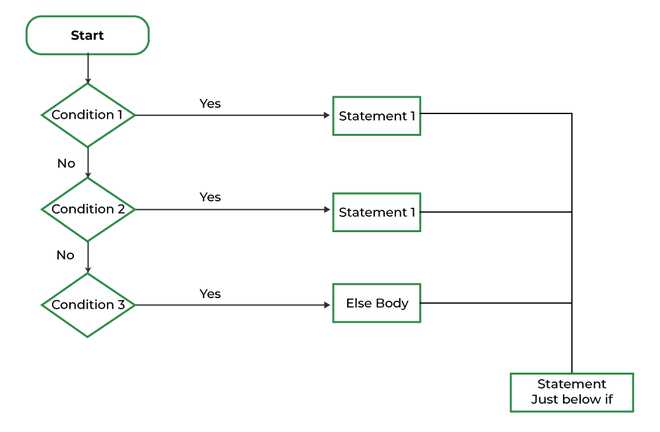# C if else if ladder

if else if ladder in C programming is used to test a series of conditions sequentially. Furthermore, if a condition is tested only when all previous if conditions in the if-else ladder are false. If any of the conditional expressions evaluate to be true, the appropriate code block will be executed, and the entire if-else ladder will be terminated.

Syntax:

```// any if-else ladder starts with an if statement only
if(condition) {

}
else if(condition) {
// this else if will be executed when condition in if is false and
// the condition of this else if is true
}
.... // once if-else ladder can have multiple else if
else { // at the end we put else

}```

## Working Flow of the if-else-if ladder:

1. The flow of the program falls into the if block.
2. The flow jumps to 1st Condition
3. 1st Condition is tested respectively:
• If the following Condition yields true, go to Step 4.
• If the following Condition yields false, go to Step 5.
4. The present block is executed. Goto Step 7.
5. The flow jumps to Condition 2.
• If the following  Condition yields true, go to step 4.
• If the following Condition yields false, go to Step 6.
6. The flow jumps to Condition 3.
• If the following Condition yields true, go to step 4.
• If the following Condition yields false, execute the else block. Goto Step 7.if else if ladder flowchart  in C

Note:  A if-else ladder can exclude else block.

Example 1: Check whether a number is positive, negative or 0

## C

 `// C Program to check whether` `// a number is positive, negative` `// or 0 using if else if ladder` `#include `   `int` `main()` `{` `    ``int` `n = 0;`   `    ``// all Positive numbers will make this` `    ``// condition true` `    ``if` `(n > 0) {` `        ``printf``(``"Positive"``);` `    ``}`   `    ``// all Negative numbers will make this` `    ``// condition true` `    ``else` `if` `(n < 0) {` `        ``printf``(``"Negative"``);` `    ``}`   `    ``// if a number is neither Positive nor Negative` `    ``else` `{` `        ``printf``(``"Zero"``);` `    ``}` `    ``return` `0;` `}`

Output

`Zero`

Example 2: Calculate Grade According to marks

## C

 `// C Program to Calculate Grade According to marks` `// using the if else if ladder` `#include ` `int` `main()` `{` `    ``int` `marks = 91;` `    ``if` `(marks <= 100 && marks >= 90)` `        ``printf``(``"A+ Grade"``);` `    ``else` `if` `(marks < 90 && marks >= 80)` `        ``printf``(``"A Grade"``);` `    ``else` `if` `(marks < 80 && marks >= 70)` `        ``printf``(``"B Grade"``);` `    ``else` `if` `(marks < 70 && marks >= 60)` `        ``printf``(``"C Grade"``);` `    ``else` `if` `(marks < 60 && marks >= 50)` `        ``printf``(``"D Grade"``);` `    ``else` `        ``printf``(``"F Failed"``);` `    ``return` `0;` `}`

Output

`A+ Grade`

Whether you're preparing for your first job interview or aiming to upskill in this ever-evolving tech landscape, GeeksforGeeks Courses are your key to success. We provide top-quality content at affordable prices, all geared towards accelerating your growth in a time-bound manner. Join the millions we've already empowered, and we're here to do the same for you. Don't miss out - check it out now!

Previous
Next